Someone's Intermediate Representation

0%

Isomorphism or invertible map will be discussed in this chapter.

## Isomorphism as Invertible Map

The crucial part of such map property is that an inverse map exists that $f: A \longrightarrow B$ has a $g$ inverse that satisfies $g \circ f \equiv 1_A$ and $f \circ g \equiv 1_B$.

A similarity between two collections can be given by choosing a map, which maps each element from the first one to the second one.

Thus isomorphism is also called invertible map. $A,B$ two objects are said to be isomorphic if an isomorphism $f:A\longrightarrow B$ exists. Properties:

• Reflexive: $A$ is isomorphic to $A$.
• Symmetric: If $A$ is isomorphic to $B$, $B$ is isomorphic to $A$.
• Transitive: If $A$ is isomorphic to $B$, $B$ is isomorphic to $C$, $A$ is isomorphic to $C$.

Thus it is obvious that $1_A$ is an isomorphism, if $f:A\longrightarrow B$ is an isomorphism, $g$ as inverse of $f$ is also an isomorphism.

It is also obvious that the inverse (if exists) of any map $f$ is unique, which means only one $g$ be $f-$inv or $f^{-1}$.

Two simple problems may occur, one is “determination“ or “extensionand the other one is “choice“ or “lifting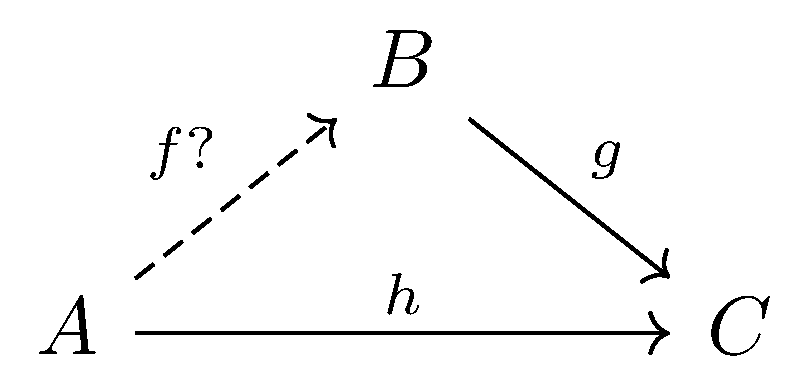### Examples

What if we have “determination“ problem with $B = \boldsymbol{1}$, which makesIt is easy to see that $g$ is a point to $C$, which means $g:\boldsymbol{1}\longrightarrow C$. Thus $h(x)$ for $x \in A$ have $h(x) = (g \circ f)(x) = g(b)$ for $b \in B \equiv \boldsymbol{1}$.

In this way, $h$ is a constant because $\forall x \in A$, $h$ maps them to a single value $g(b)$ on $C$.

Another example here is on “choice“ problem with $A = C$, which makesTo make things easier, we give a small example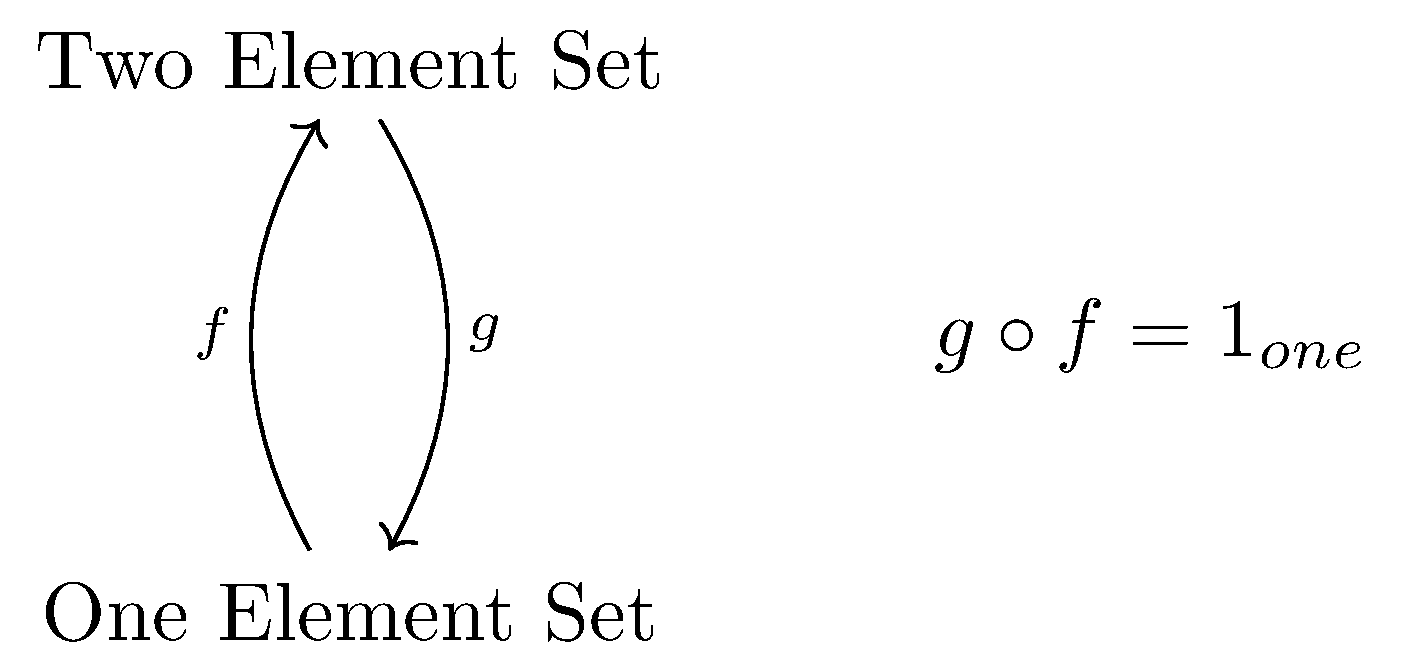It is not hard to see that $g$ is constant and $f$ has two choices. The only way for $f$ existence is that $|B| \ge |A|$ and every element in $C$ is mapped.

## Retraction, Section

Retraction can be defined if $f:A \longrightarrow B$, a retraction for $f$ is a map $r :B \longrightarrow A$ for which $r \circ f = 1_A$. The determination problem can be simplified in a way shown in following pictures.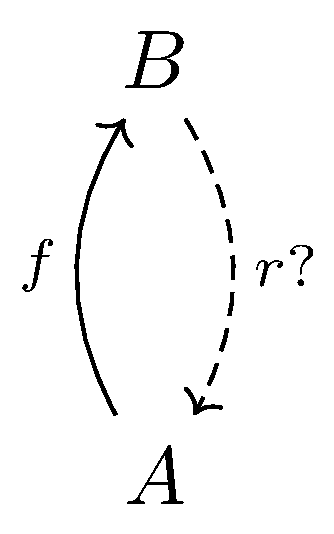Section can be defined if $f : A \longrightarrow B$, a section for $f$ is a map $s: B\longrightarrow A$ for which $f \circ s = 1_B$. The choice problem can be simplified in a way shown in following pictures.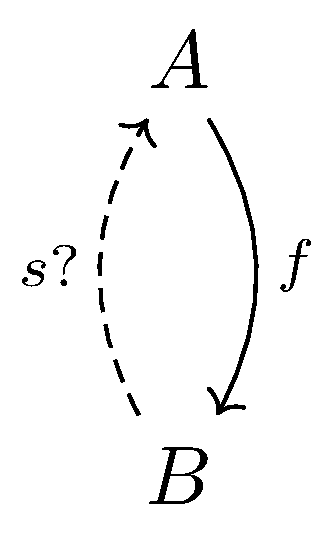It is not hard to see that, if $f:A\longrightarrow B$ has a section $s:B\longrightarrow A$, then $\forall T$ and $\forall m:T\longrightarrow B, \exists x:T\longrightarrow X$ that $f \circ x = m$.The dual version is: If $f:A\longrightarrow B$ has a retraction $r:B\longrightarrow A$, then $\forall T, \forall m: A\longrightarrow T, \exists x: B\longrightarrow T$ that $x \circ f = m$.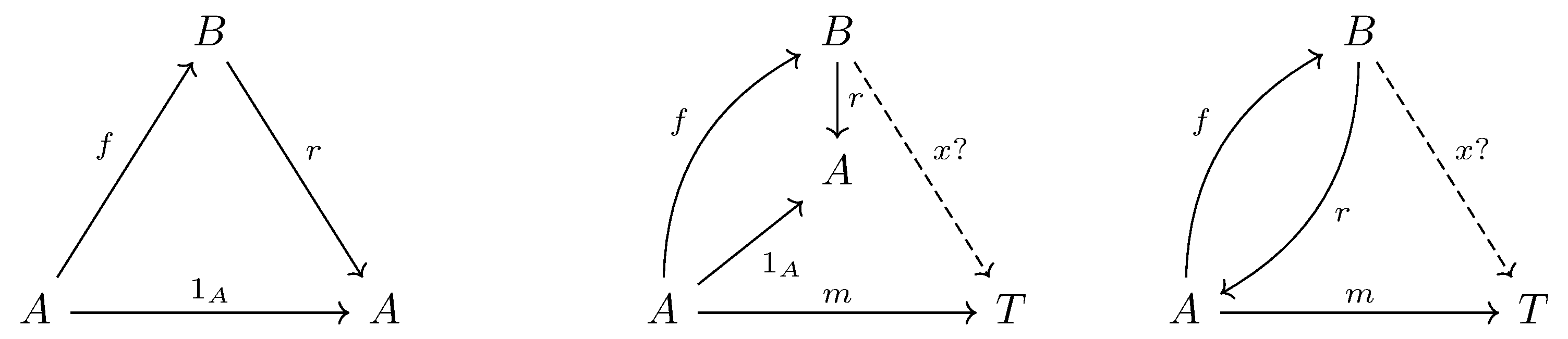## Epimorphism, Monomorphism, Idempotent

Suppose $f:A\longrightarrow B$ has a retraction, then $\forall T, \forall x_1, x_2:T\longrightarrow A$, if $f \circ x_1 = f\circ x_2$, then $x_1 = x_2$.

A map $f$ satisfying this conclusion is said to be injective for maps from $T$. If $\forall T$ is satisfied, then $f$ is injective, or is a monomorphism.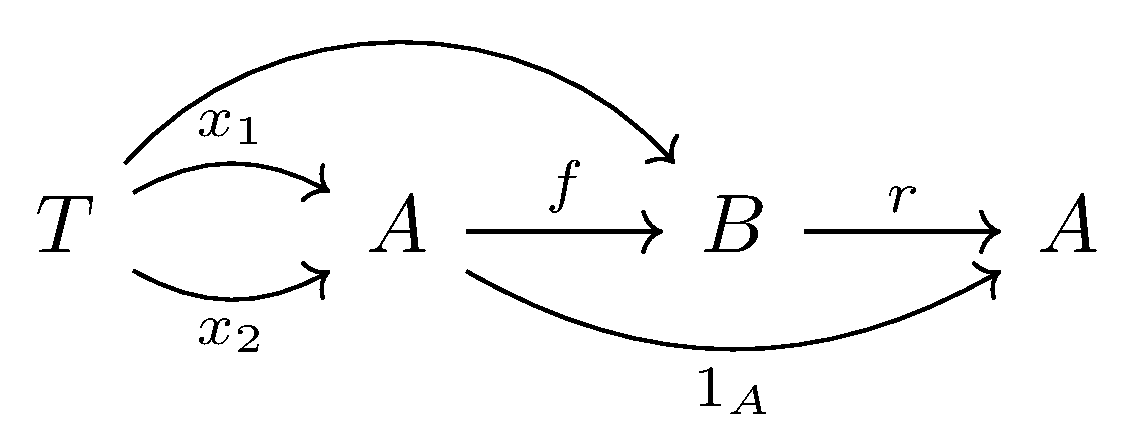The dual version is that $f :A\longrightarrow B$ has a section, then $\forall T, \forall x_1, x_2:B \longrightarrow T$, if $x_1 \circ f = x_2 \circ f$, then $x_1 = x_2$.

A map $f$ with cancellation property ($x_1 \circ f = x_2 \circ f$ then $x_1 = x_2$) for any $T$ is called epimorphism.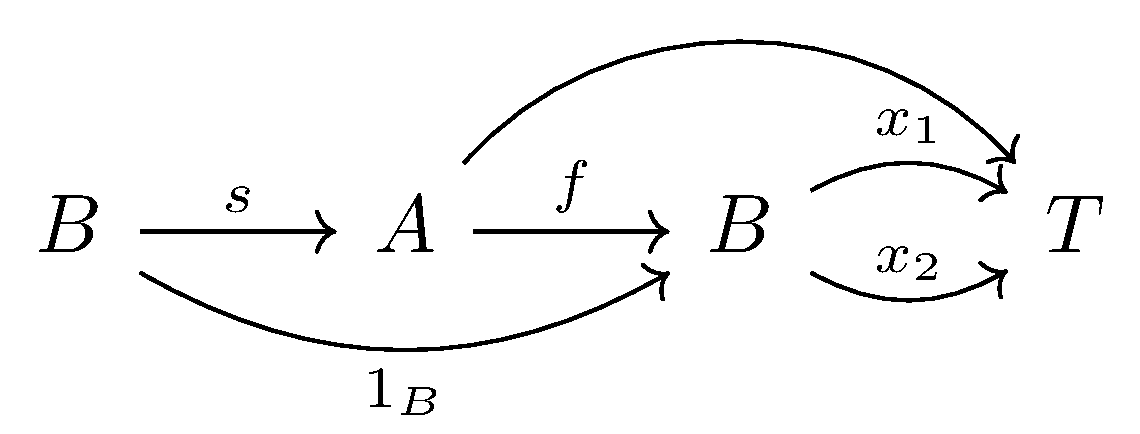Both epimorphism and monomorphism are cancellation properties.

An endomap $e$ is called idempotent if $e \circ e = e$.

If $f: A\longrightarrow B$ has both a retraction $r$ and a section $s$, then $r = s$.

## Isomorphism, Automorphism

We can rephrase isomorphism with retraction and section:

A map $f$ is called an isomorphism if $\exists f^{-1}$ which is both a retraction and a section for $f$ that $f \circ f^{-1} = 1_B$ and $f^{-1} \circ f = 1_A$.

A map, which is an isomorphism and an endomap at a same time, is an automorphism.

In general, if any isomorphism exists for $A\longrightarrow B$, there are the same number of them as there are automorphism of $A$, and this can be proved without counting.

Let $Aut(A)$ be the set of all automorphisms of $A$ and $Isom(A,B)$ be the set of all isomorphisms of $A\longrightarrow B$. We just need to construct isomorphism between those two sets.

If $f : A\longrightarrow B$ is an isomorphism, then $F : Aut(A)\longrightarrow Isom(A,B)$ can be constructed by $F(\alpha) = f\circ \alpha$ for any automorphism $\alpha$ on $A$.$F(\alpha) \in Isom(A,B)$, and we want to show $F$ itself is an isomorphism, by constructing $S = F^{-1}: Isom(A,B)\longrightarrow Aut(A)$.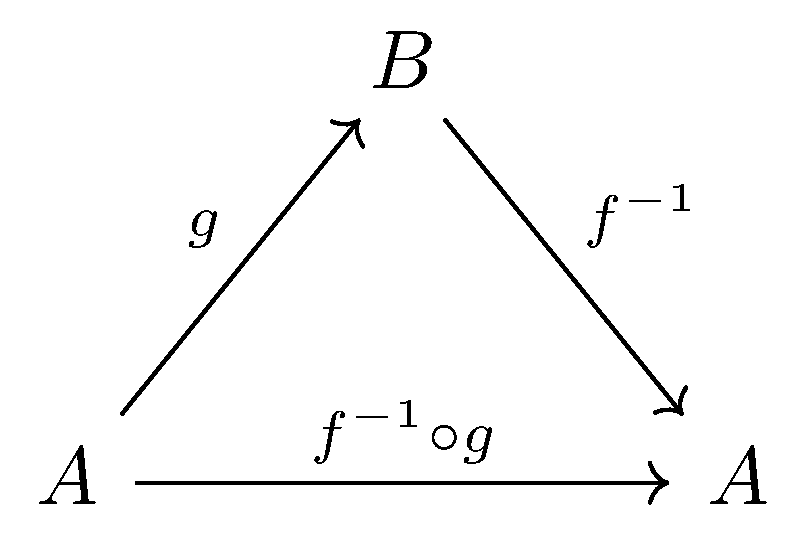Thus, $S(g) = f^{-1} \circ g$.
\begin{aligned} (F \circ S)(g) &= F(S(g))= F(f^{-1}\circ g) = f \circ (f^{-1}\circ g) = g\newline (S \circ F)(\alpha) &= S(F(\alpha)) =S (f\circ \alpha) = f^{-1} \circ(f\circ \alpha) = \alpha \end{aligned}
We can see that $F\circ S = 1_{Isom(A,B)}$ and $S\circ F = 1_{Aut(A)}$.

### Automorphism, or Permutation

An automorphism in category of sets is traditionally called a permutation, suggesting that it shifts elements of its sets around in a specified way.

A category of permutation has an object defined with a set $A$ and an automorphism $\alpha$. Thus an object is defined as $A^{\Large\circlearrowright_\alpha}$.

A map from $A^{\Large\circlearrowright_\alpha}$ to $B^{\Large\circlearrowright_\beta}$ is a map of $f : A\longrightarrow B$ which preserve the automorphisms $\alpha$ and $\beta$ in a sense that $f \circ \alpha = \beta\circ f$.If $g$ is a map from $B^{\Large\circlearrowright_\beta}$ to $C^{\Large\circlearrowright_\gamma}$, and we want to compose $f$ and $g$, the natural thing is to compose them as $A \overset{f}{\longrightarrow} B \overset{g}{\longrightarrow} C$.

The verification is shown in the following commutative diagram, which indicates $g \circ f \circ \alpha = g \circ \beta\circ f = \gamma \circ g \circ f$.